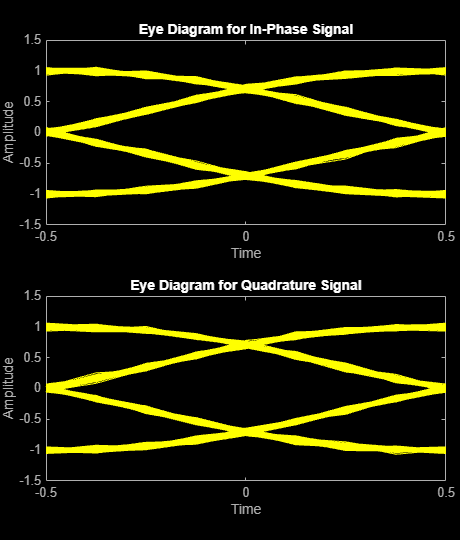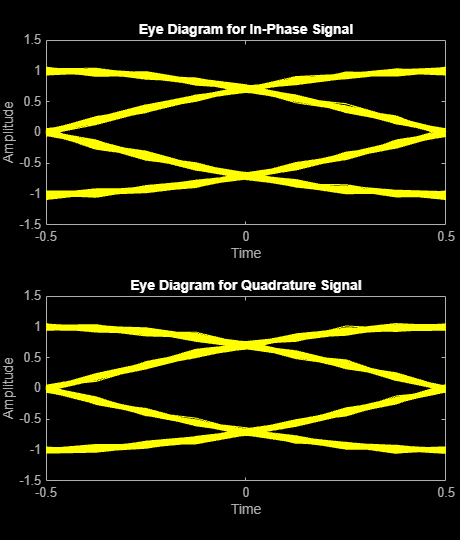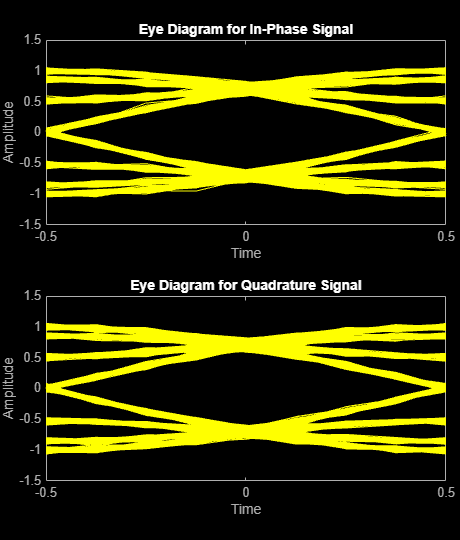# comm.MSKModulator

Modulate using MSK method

## Description

The `comm.MSKModulator` System object™ modulates using the minimum shift keying method. The output is a baseband representation of the modulated signal. For more information, see Algorithms.

To modulate a signal using minimum shift keying:

1. Create the `comm.MSKModulator` object and set its properties.

2. Call the object with arguments, as if it were a function.

## Creation

### Syntax

``mskmodulator = comm.MSKModulator``
``mskmodulator = comm.MSKModulator(Name=Value)``

### Description

example

````mskmodulator = comm.MSKModulator` creates a modulator System object that modulates the input signal using the minimum shift keying (MSK) modulation method.```

example

````mskmodulator = comm.MSKModulator(Name=Value)` sets Properties using one or more name-value arguments. For example, `mskmodulator = comm.MSKModulator(InitialPhaseOffset=pi/2)` specifies an initial phase of `pi/2` radians for the modulated waveform.```

## Properties

expand all

Unless otherwise indicated, properties are nontunable, which means you cannot change their values after calling the object. Objects lock when you call them, and the `release` function unlocks them.

If a property is tunable, you can change its value at any time.

Option to provide input in bits, specified as a numeric or logical `0` (`false`) or `1` (`true`).

• When you set this property to `false`, the input to the System object call requires a double-precision or signed integer data type column vector with values of `-1` or `1`.

• When you set this property to `true`, the input to the System object call requires a double-precision or logical data type column vector of `0`s and `1`s.

Data Types: `logical`

Initial phase offset of the modulated waveform in radians, specified as a numeric scalar.

Number of samples per output symbol, specified as a positive integer. The number of samples per symbol represents the upsampling factor from input samples to output samples.

Data Types: `double`

Output data type, specified as either `"double"` or `"single"`.

## Usage

### Syntax

``y = mskmodulator(x)``

### Description

````y = mskmodulator(x)` applies MSK modulation to the input data and returns the modulated MSK baseband signal.```

### Input Arguments

expand all

Input data, specified as an integer or column vector of integers or bits.

The setting of the `BitInput` property determines the interpretation of the input data. If the property is set to `false`, the input must take values of 1 or -1.

Data Types: `double` | `logical`

### Output Arguments

expand all

MSK-modulated baseband signal, returned as a column vector.

The length of the vector is equal to the number of input samples times the `SamplesPerSymbol` property. For more information about the output data type, see the `OutputDataType` property.

Data Types: `single` | `double`
Complex Number Support: Yes

## Object Functions

To use an object function, specify the System object as the first input argument. For example, to release system resources of a System object named `obj`, use this syntax:

`release(obj)`

expand all

 `step` Run System object algorithm `release` Release resources and allow changes to System object property values and input characteristics `reset` Reset internal states of System object

## Examples

collapse all

Create an MSK modulator, an AWGN channel, and an MSK demodulator. Use a phase offset of $\pi /4$.

```mskmodulator = comm.MSKModulator( ... BitInput=true, ... InitialPhaseOffset=pi/4); awgn = comm.AWGNChannel( ... NoiseMethod='Signal to noise ratio (SNR)', ... SNR=0); mskdemodulator = comm.MSKDemodulator( ... BitOutput=true, ... InitialPhaseOffset=pi/4);```

Create an error rate calculator. Account for the delay caused by the Viterbi algorithm.

` ber = comm.ErrorRate(ReceiveDelay=mskdemodulator.TracebackDepth);`

Transmit 100 3-bit words.

``` for counter = 1:100 data = randi([0 1],300,1); modSignal = mskmodulator(data); noisySignal = awgn(modSignal); receivedData = mskdemodulator(noisySignal); errorStats = ber(data, receivedData); end fprintf('Error rate = %f\nNumber of errors = %d\n', ... errorStats(1), errorStats(2))```
```Error rate = 0.000000 Number of errors = 0 ```

Map binary sequences of zeros and ones to the output of an MSK modulator. This mapping also applies for GMSK modulation.

Create an MSK modulator that accepts binary inputs and has a samples per symbol value of 1.

`mskmodulator = comm.MSKModulator(BitInput=true, SamplesPerSymbol=1);`

Create an input sequence of all zeros. Modulate the sequence.

```x = zeros(5,1); y = mskmodulator(x)```
```y = 5×1 complex 1.0000 + 0.0000i 0.0000 - 1.0000i -1.0000 - 0.0000i -0.0000 + 1.0000i 1.0000 + 0.0000i ```

Determine the phase angle for each point. Use the `unwrap` function to show the trend.

`theta = unwrap(angle(y))`
```theta = 5×1 0 -1.5708 -3.1416 -4.7124 -6.2832 ```

A sequence of zeros causes the phase to shift by $-\pi /2$ between samples.

Reset the modulator. Modulate an input sequence of all ones.

```reset(mskmodulator) x = ones(5,1); y = mskmodulator(x)```
```y = 5×1 complex 1.0000 + 0.0000i 0.0000 + 1.0000i -1.0000 + 0.0000i -0.0000 - 1.0000i 1.0000 + 0.0000i ```

Determine the phase angle for each point. Use the `unwrap` function to show the trend.

`theta = unwrap(angle(y))`
```theta = 5×1 0 1.5708 3.1416 4.7124 6.2832 ```

A sequence of ones causes the phase to shift by $+\pi /2$ between samples.

Compare Gaussian minimum shift keying (GMSK) and minimum shift keying (MSK) modulation schemes by plotting the eye diagram for GMSK with different pulse lengths and for MSK.

Set the samples per symbol variable.

`sps = 8;`

Generate random binary data.

`data = randi([0 1],1000,1);`

Create GMSK and MSK modulators that accept binary inputs. Set the `PulseLength` property of the GMSK modulator to `1`.

```gmskMod = comm.GMSKModulator('BitInput',true,'PulseLength',1, ... 'SamplesPerSymbol',sps); mskMod = comm.MSKModulator('BitInput',true,'SamplesPerSymbol',sps);```

Modulate the data using the GMSK and MSK modulators.

```modSigGMSK = gmskMod(data); modSigMSK = mskMod(data);```

Pass the modulated signals through an AWGN channel having an SNR of `30` dB.

```rxSigGMSK = awgn(modSigGMSK,30); rxSigMSK = awgn(modSigMSK,30);```

Use the `eyediagram` function to plot the eye diagrams of the noisy signals. With the GMSK pulse length set to `1`, the eye diagrams are nearly identical.

`eyediagram(rxSigGMSK,sps,1,sps/2)``eyediagram(rxSigMSK,sps,1,sps/2)`Set the `PulseLength` property for the GMSK modulator object to `3`. Because the property is nontunable, the object must be released first.

```release(gmskMod) gmskMod.PulseLength = 3;```

Generate a modulated signal using the updated GMSK modulator object and pass it through the AWGN channel.

```modSigGMSK = gmskMod(data); rxSigGMSK = awgn(modSigGMSK,30);```

With continuous phase modulation (CPM) waveforms, such as GSMK, the waveform depends on values of the previous symbols as well as the present symbol. Plot the eye diagram of the GMSK signal to see that the increased pulse length results in an increase in the number of paths in the eye diagram.

`eyediagram(rxSigGMSK,sps,1,sps/2)`Experiment by changing the `PulseLength` parameter of the GMSK modulator object to other values. If you set the property to an even number, you should set `gmskMod.InitialPhaseOffset` to `pi/4` and update the offset argument of the `eyediagram` function from `sps/2` to `0` for a better view of the modulated signal. In order to more clearly view the Gaussian pulse shape, you must use scopes that display the phase of the signal, as described in the View CPM Phase Tree Using Simulink example.

## Algorithms

Differentially encoded minimum shift keying modulation uses pulse shaping to smooth the phase transitions of the modulated signal. The function q(t) is the phase response obtained from the frequency pulse, g(t), through this relation:$q\left(t\right)={\int }_{-\text{\hspace{0.17em}}\infty }^{t}g\left(t\right)dt$.

The specified frequency pulse shape corresponds to this rectangular pulse shape expression for g(t).

Pulse ShapeExpression
`Rectangular`$g\left(t\right)=\left\{\begin{array}{cc}\frac{1}{2LT},& 0\le t\le LT\\ 0& \text{otherwise}\end{array}$

• L is the main lobe pulse duration in symbol intervals.

• The duration of the pulse, LT, is the pulse length in symbol intervals.

## Version History

Introduced in R2012a